# cs231n - Neural Networks

## Introduction to Neural Networks [slides]

Posted by kevin on November 9, 2019

## Neural Networks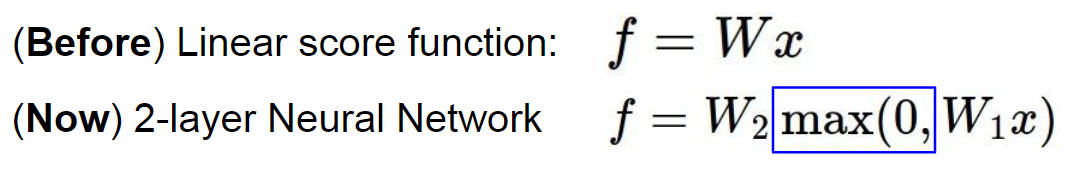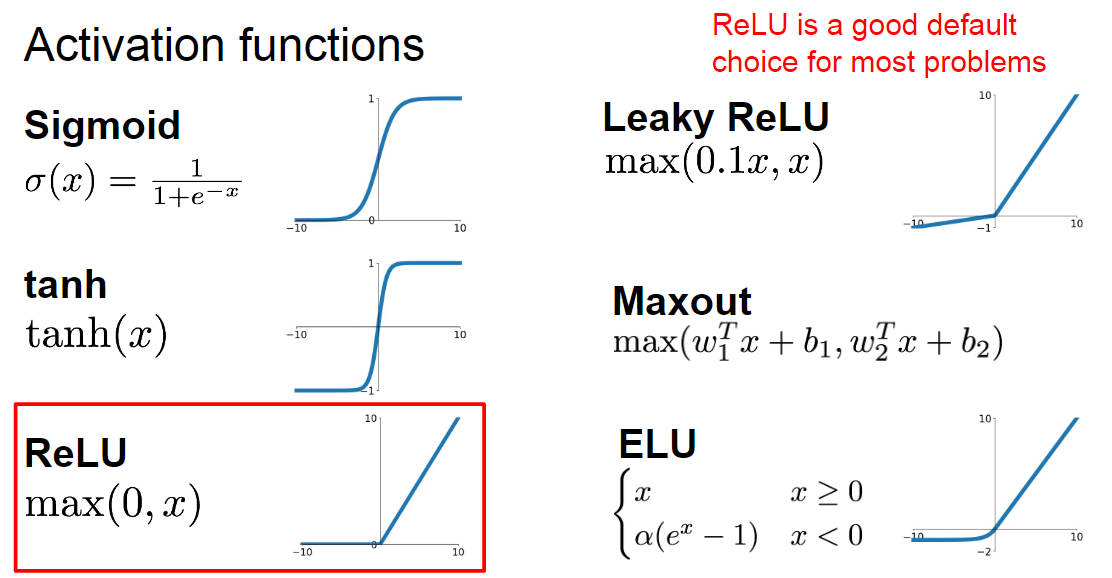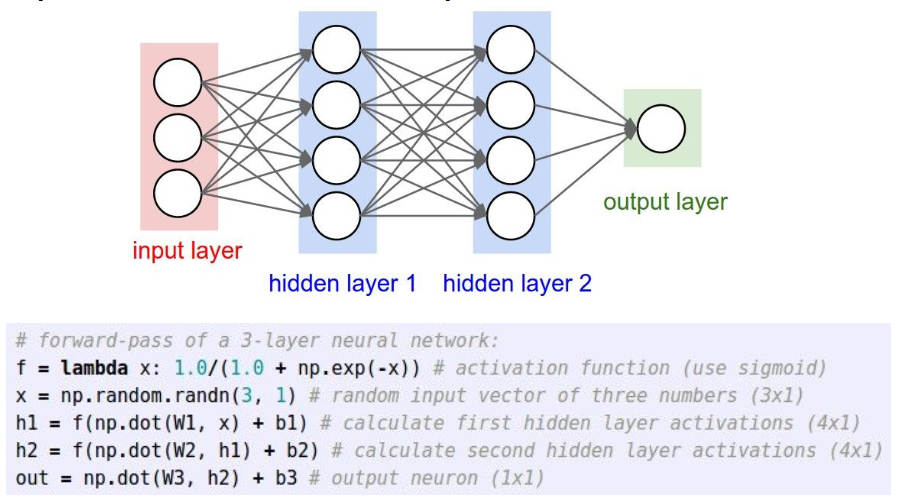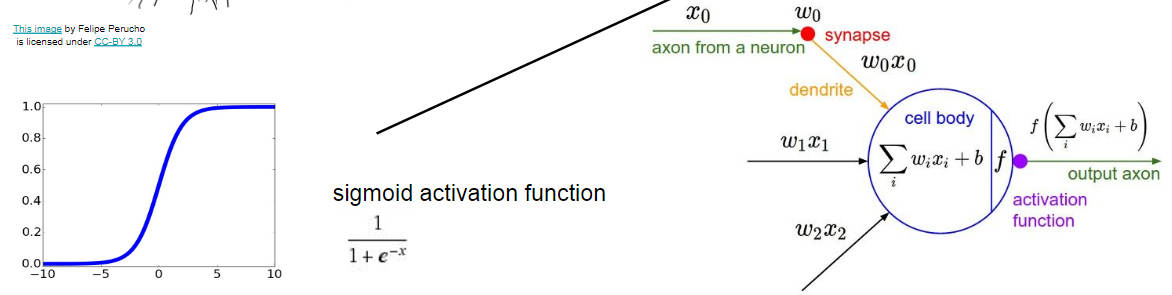## How to compute gradients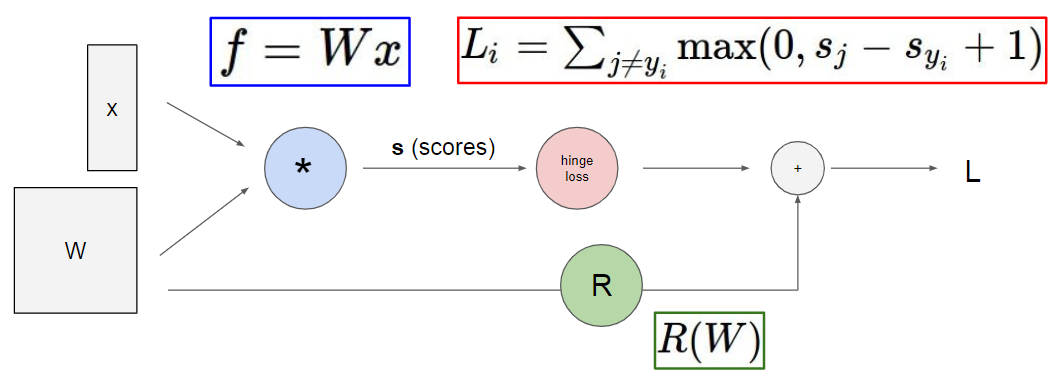### Backpropagation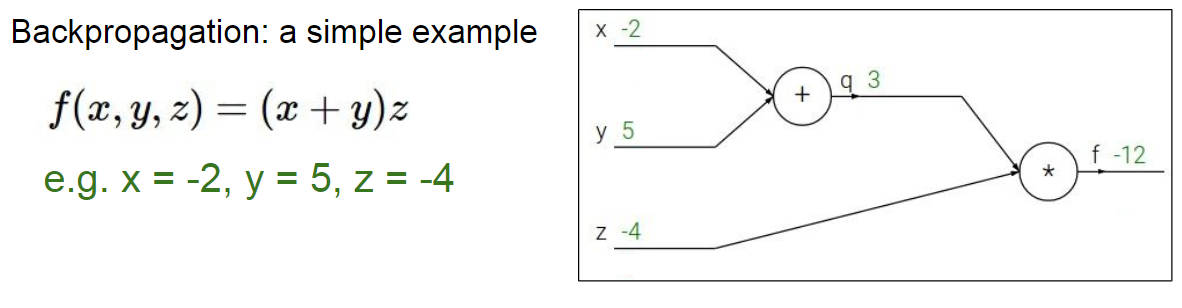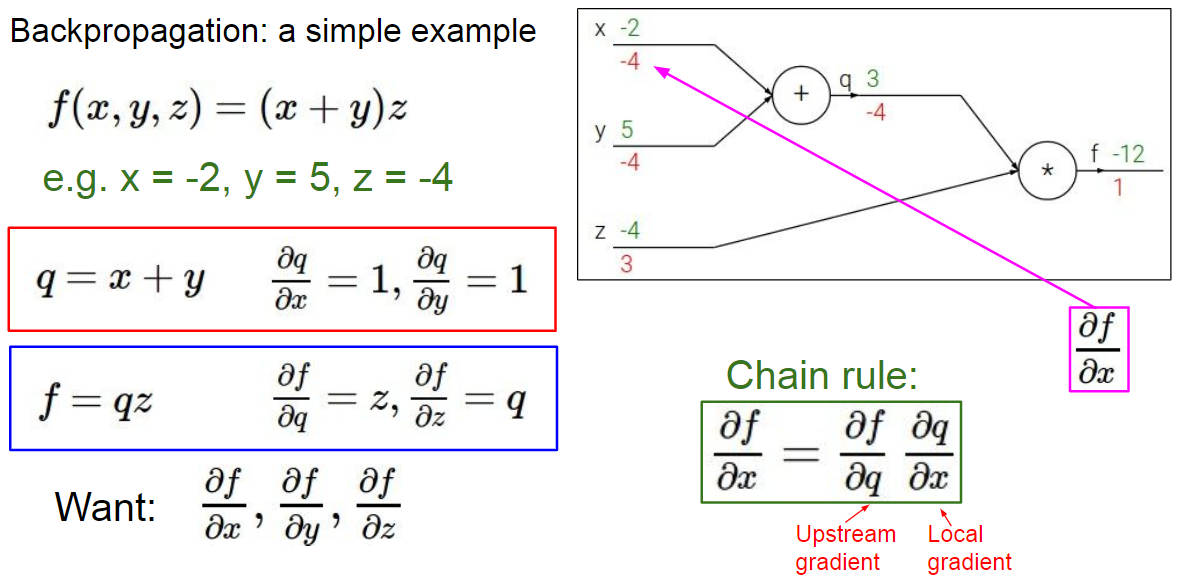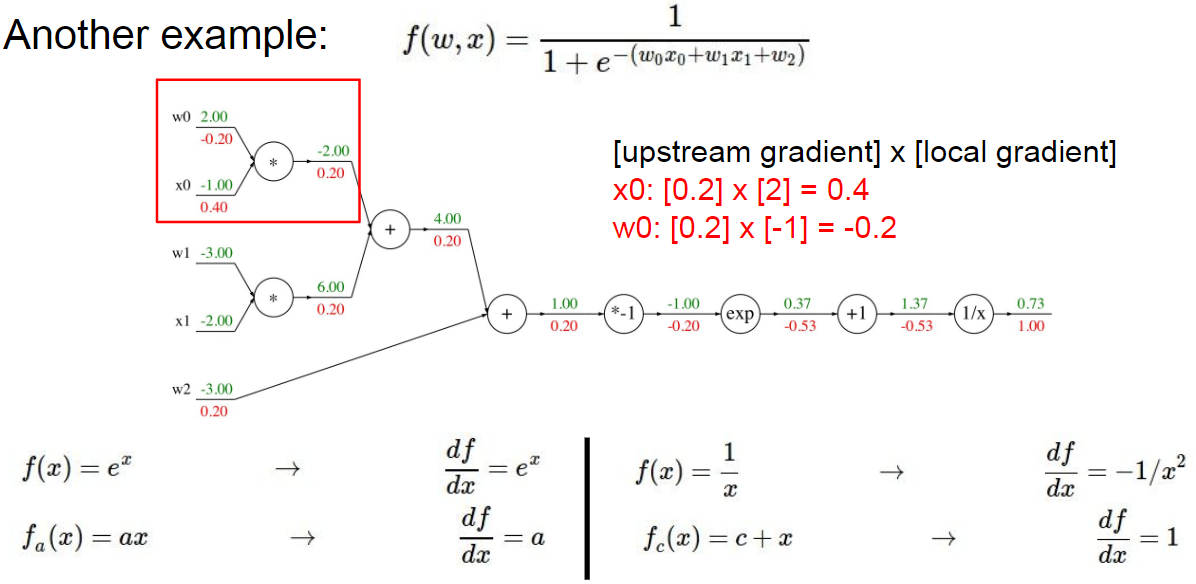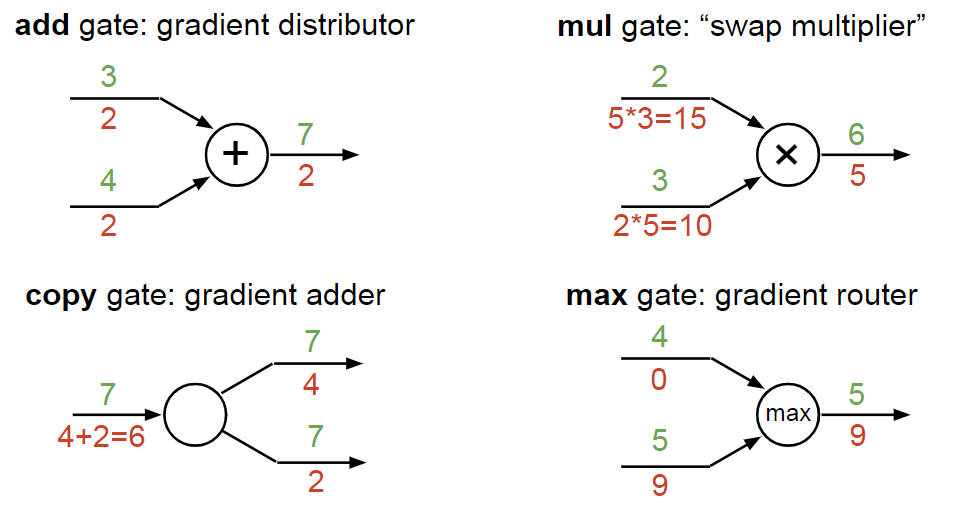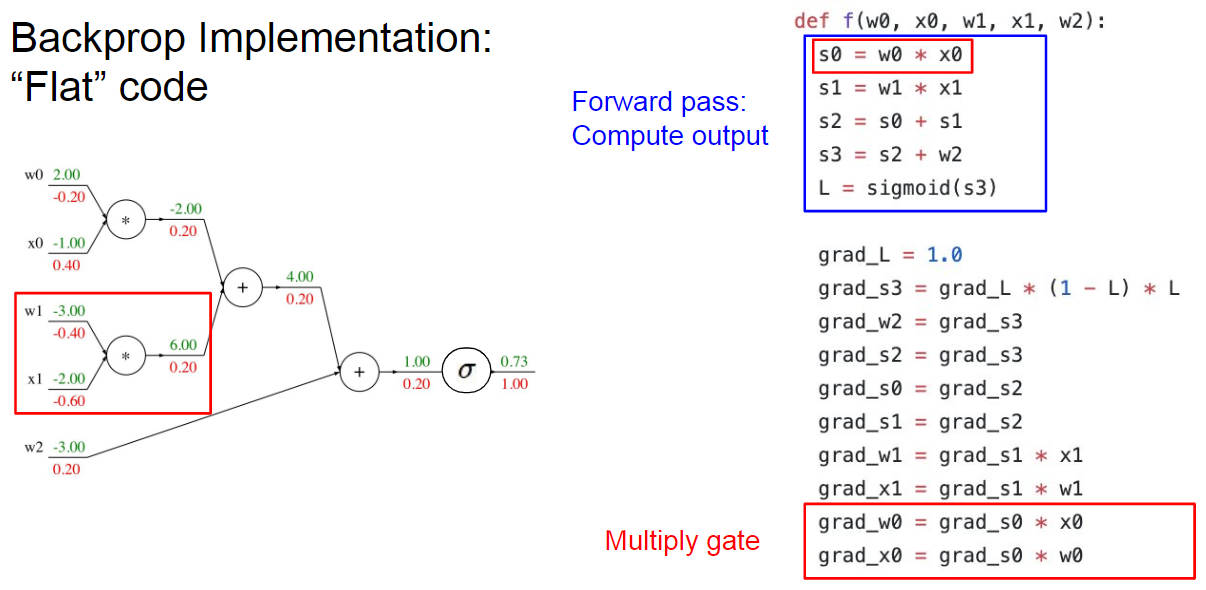### Backprop with vector-valued functions

# forward pass
W = np.random.randn(5, 10)
X = np.random.randn(10, 3)
D = W.dot(X)

# now suppose we had the gradient on D from above in the circuit
dD = np.random.randn(*D.shape) # same shape as D
dW = dD.dot(X.T) #.T gives the transpose of the matrix
dX = W.T.dot(dD)